# SAS/ETS Examples

## Fitting a Capital Asset Pricing Model

Contents | SAS Program

# Overview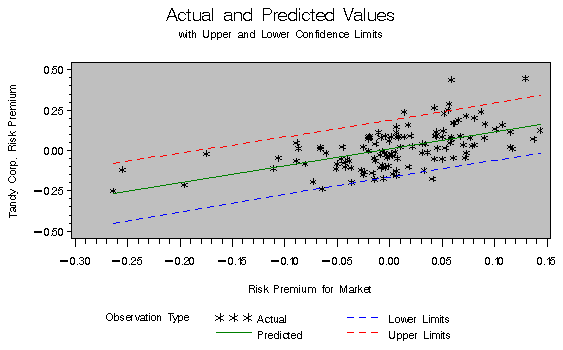The Capital Asset Pricing Model (CAPM) is one of the most common methods of relating the sensitivity of an individual company's stock return to the return of the market as a whole. Specifically, the CAPM linear relationship can be written aswhere rj, rf, and rm are returns to security j, the risk-free asset and the market return, respectively. The term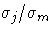is the ratio of the standard deviations of returns on security j to the market.

A full development of the CAPM can be found in any introductory finance textbook (for example, Brealey and Myers 1988), and so is omitted here. There are, however, several econometric issues involved in the more general model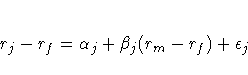where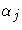is an intercept term, andis an IID stochastic disturbance term with mean zero and variance.

Two straightforward tests of this model are

1. a test of the null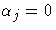against the alternative that it is not equal to 0. A rejection would cast some doubt on the theoretical model asis assumed to be 0 in the first equation.
2. a test of the null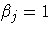against the alternative that it is not equal to 1. Failure to reject would imply that the movement of asset prices of a particular company is the same as that of the market as a whole.

This example uses stock returns from the Tandy Corporation to estimate a CAPM and perform some general tests of the model.

# Analysis

The CAPM relates the sensitivity of an individual company's stock returns to the returns of the market as a whole. Estimating a model for a particular firm requires data on the market rate of return (typically a composite index such as the S&P 500 or the Dow Jones Industrial Average), the risk-free rate of return (usually a short-term Treasury bill), and stock returns from the company of interest.

The data for this example consist of monthly observations from January 1978 through December 1987 on the market return, the risk-free rate, and the return on the Tandy Corporation's common stock. Using a SAS DATA step, enter the data and create two new variables, R_TANDY = TANDY - R_F and R_MKT = R_M - R_F, that correspond to the risk premiums for the Tandy Corporation and the Market. A risk premium is the excess return of a security over the risk-free rate or, rather, the extra return that investors require for bearing risk.

   data tandy;
input r_m r_f tandy @@;
r_tandy = tandy - r_f;
r_mkt = r_m -r_f;
label r_m='Market Rate of Return'
r_f='Risk-Free Rate of Return'
tandy='Rate of Return for Tandy Corporation'
datalines;
-.045 .00487 -.075
.063 .00491  .055
.071 .00528  .194
... more data ...
;
run;


It is always a good idea to look at the data to be sure that it was input as expected. A simple plot allows for a visual assessment of the appropriateness of the proposed model. The following code uses the GPLOT procedure to plot the risk premium of the Tandy Corporation against the risk premium of the Market.

   proc gplot data=tandy;
plot r_tandy * r_mkt / haxis=axis1 hminor=4 cframe=ligr
vaxis=axis2 vminor=4;
symbol1 c=blue v=star;
axis1 order=(-0.3 to 0.2 by 0.1);
axis2 label=(angle=90 'Tandy Corp. Risk Premium')
order=(-0.4 to 0.6 by 0.2);
title 'TANDY Corporation CAPM Example';
title3'Tandy Corporation versus the Market';
run;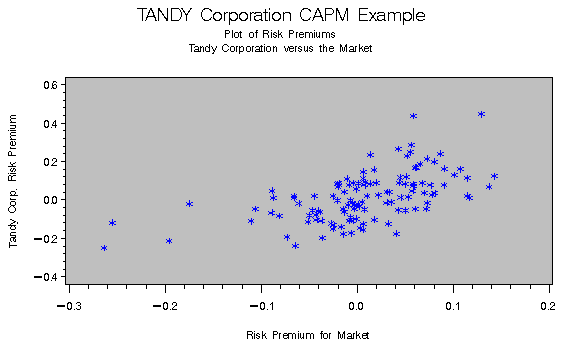The plot of the Tandy data set above indicates that there is a positive relationship between the risk premium of the market and the risk premium of the Tandy Corporation's stock. This positive relationship seems sensible: as the market return increases, so should the return of most stocks. Based on this visual evidence, a linear model between R_TANDY and R_MKT is not unreasonable.

The AUTOREG procedure specifies a linear regression of R_TANDY on R_MKT. Note that a constant term is automatically included in the model unless the NOINT option is specified. The DWPROB option requests the Durbin-Watson test for autocorrelation of the residuals. The TEST statement enables you to perform hypothesis tests on parameter estimates; the following TEST statement is requesting a test that the coefficient of R_MKT is equal to 1. In addition, the OUTPUT data set TANDYOUT contains the predicted values of R_TANDY, the residuals of the regression, and the upper and lower bounds of the 90% confidence interval.

   proc autoreg data=tandy;
model r_tandy = r_mkt / dwprob;
test r_mkt = 1;
output out=tandyout p=p r=r ucl=u lcl=l alphacli=.10;
title2;
title3;
run;


 The AUTOREG Procedure

 Dependent Variable r_tandy Risk Premium for Tandy Corporation

 Ordinary Least Squares Estimates SSE 1.31740165 DFE 118 MSE 0.01116 Root MSE 0.10566 SBC -191.29942 AIC -196.8744 Regress R-Square 0.3191 Total R-Square 0.3191 Durbin-Watson 1.9334 Pr < DW 0.3587 Pr > DW 0.6413

 Variable DF Estimate Standard Error t Value Approx Pr > |t| Variable Label Intercept 1 0.0107 0.009698 1.10 0.2740 r_mkt 1 1.0500 0.1412 7.44 <.0001 Risk Premium for Market

 Test 1 Source DF Mean Square F Value Pr > F Numerator 1 0.001400 0.13 0.7239 Denominator 118 0.011164

The output provides information about the appropriateness of the model chosen. The parameter estimate for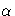, the intercept, is not significantly different from 0. The estimate for, the coefficient of R_MKT, is 1.05 and is highly significant. So, not altogether unexpectedly, it seems that the market risk premium is a factor in the risk premium for the Tandy Corporation.

The F-statistic for the test that the coefficient of R_MKT is equal to one is 0.1254 with an associated p-value of 0.724, implying that there is little evidence that this coefficient is different from 1.0. Thus there is little evidence that the returns of the Tandy stock were any more or less volatile than the returns of the market.

R2 measures the proportion of the total variation of Y explained by the regression of Y on the independent variable, X. The R2 value of 0.319 means that about 32% of the variation in the risk premium of Tandy Corporation stock can be explained by the risk premium of the market. Or, put another way, 68% of the risk premium of Tandy stock is firm specific.

The Durbin-Watson d statistic tests for autocorrelation and lack of independence of residuals, which is a common problem in time series data. The d statistic ranges from 0 to 4. A value close to 2.0 indicates that you cannot reject the null hypothesis of no autocorrelation. The calculated value of the d statistic in the CAPM for the Tandy Corporation is 1.933, and it has a p-value of 0.359. This value indicates that, for this model, you cannot reject the null hypothesis of independent (nonautocorrelated) residuals at typically chosen levels of significance.

Using PROC GPLOT, you can investigate the residuals for patterns that would indicate a violation of the underlying assumptions of the model.

   proc gplot data=tandyout;
plot r * r_mkt / haxis=axis1 hminor=4 cframe=ligr
vaxis=axis2 vminor=4
vref=0.0;
symbol1 c=green v=star;
axis1 order=(-0.3 to 0.2 by 0.1);
axis2 label=(angle=90 'Tandy Corp. Risk Premium')
order=(-0.3 to 0.4 by 0.1);
title 'TANDY Corporation CAPM Example';
title2'OLS Residuals versus Market Risk Premium';
run;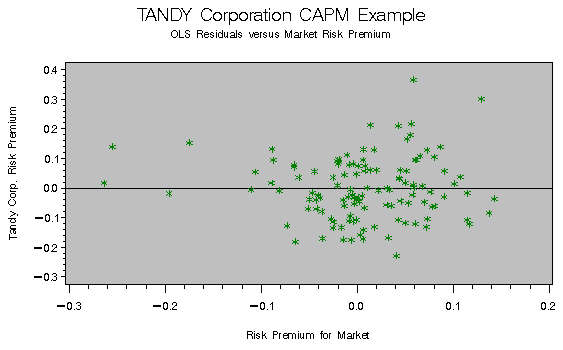This residual plot does not reveal any obvious patterns; the residuals appear randomly distributed about the reference line.

# References

Berndt, Ernst R. (1991), The Practice of Econometrics: Classic and Contemporary, New York: Addison-Wesley.

Brealey, Richard A. and Myers, Stewart C. (1988), Principles of Corporate Finance, Third Edition, New York: McGraw-Hill.

SAS Institute Inc. (1993), SAS/ETS Software: Applications Guide 2, Version 6, First Edition: Econometric Modeling, Simulation, and Forecasting, Cary, NC: SAS Institute Inc., 15-30.